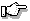# ecvt(), _ecvt()

convert a floating-point number into a string

## Synopsis:

```#include <stdlib.h>
char *ecvt( double value,
int ndigits,
int *dec,
int *sign );
char *_ecvt( double value,
int ndigits,
int *dec,
int *sign );```

## Description:

The ecvt() function converts the floating-point number value into a character string. The parameter ndigits specifies the number of significant digits desired. The converted number is rounded to ndigits of precision.

The character string contains only digits, and is terminated by a NULL character. The integer pointed to by dec will be filled in with a value indicating the position of the decimal point, relative to the start of the string of digits. A zero or negative value indicates that the decimal point lies to the left of the first digit.

The integer pointed to by sign will be 0 if the number is positive, and nonzero if the number is negative.

The _ecvt() function is identical to ecvt(); use _ecvt() for ANSI/ISO naming conventions.

## Returns:

The ecvt() function returns a pointer to a static buffer containing the converted string of digits.The functions ecvt() and fcvt() both use the same static buffer.

## Examples:

```#include <stdio.h>
#include <stdlib.h>

void main()
{
char *str;
int  dec, sign;

str = ecvt( 123.456789, 6, &dec, &sign );
printf( "str=%s, dec=%d, sign=%d\n",
str, dec, sign );
}```

produces the output:

`str=123457, dec=3, sign=0`

## Classification:

WATCOM

_ecvt() conforms to ANSI/ISO naming conventions.

Safety:
Interrupt handler No
Signal handler No